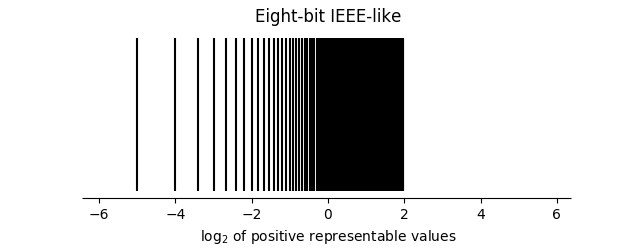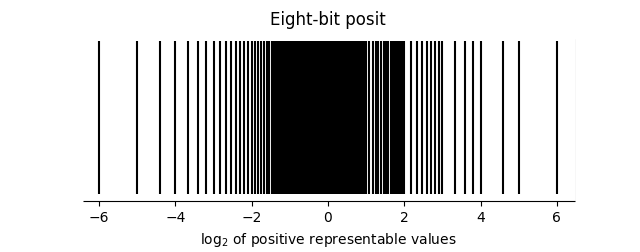# Comparing range and precision of IEEE and posit

The IEEE standard 754-2008 defines several sizes of floating point numbers—half precision (binary16), single precision (binary32), double precision (binary64), quadruple precision (binary128), etc.—each with its own specification. Posit numbers, on the other hand, can be defined for any number of bits. However, the IEEE specifications share common patterns so that you could consistently define theoretical IEEE numbers that haven’t actually been specified, making them easier to compare to posit numbers.

An early post goes into the specification of posit numbers in detail. To recap briefly, a posit<nes> number has n bits, a maximum of es of which are devoted to the exponent. The bits are divided into a sign bit, regime bits, exponent bits, and fraction bits. The sign bit is of course one bit, but the other components have variable lengths. We’ll come back to posits later for comparison.

## IEEE floating point range and precision

We will denote a (possibly hypothetical) IEEE floating point number as ieee<nes> to denote one with n total bits and (exactly) es exponent bits. Such a number has one sign bit and n – es -1 significand bits. Actual specifications exist for ieee<16, 5>, ieee<32, 8>, ieee<64, 11>, and ieee<128, 15>.

The exponent of a posit number is simply represented as an unsigned integer. The exponent of an IEEE floating point number equals the exponent bits interpreted as an unsigned integers minus a bias.So the biases for half, single, double, and quad precision floats are 15, 127, 1023, and 65535 respectively. We could use the formula above to define the bias for a hypothetical format not yet specified, assuming the new format is consistent with existing formats in this regard.

The largest exponent, emax is 2es-1 – 1 (also equal to the bias), and the smallest (most negative) exponent is emin = 2 – 2es-1. This accounts for 2es-1 – 2 possible exponents. The two remaining possibilities consist of all 1’s and all 0’s, and are reserved for special use. They represent, in combination with sign and signifcand bits, special values ±0, ±∞, NaN, and denomalized numbers. (More on denormalized numbers shortly.)

The largest representable finite number has the maximum exponent and a significand of all 1’s. Its value is thuswhere s is the number of significand bits. And so the largest representable finite number is just slightly less thanWe’ll use this as the largest representable value when calculating dynamic range below.

The smallest representable normalized number (normalized meaning the signifcand represents a number greater than or equal to 1) isHowever, it is possible to represent smaller values with denomalized numbers. Ordinarily the significand bits fff… represent a number 1.fff… But when the exponent bit pattern consists of all 0’s, the significand bits are interpreted as 0.fff… This means that the smallest denormalized number has a significand of all o’s except for a 1 at the end. This represents a value ofwhere again s is the number of significand bits.

The dynamic range of an ieee<nes> number is the log base 10 of the ratio of the largest to smallest representable numbers, smallest here including denormalized numbers.## IEEE float and posit dynamic range at comparable precision

Which posit number should we compare with each IEEE number? We can’t simply compare ieee<nes> with posit<nes>. The value n means the same in both cases: the total number of bits. And although es does mean the number of exponent bits in both cases, they are not directly comparable because posits also have regime bits that are a special kind of exponent bits. In general a comparable posit number will have a smaller es value than its IEEE counterpart.

One way to compare IEEE floating point numbers and posit numbers is to chose a posit number format with comparable precision around 1. See the first post on posits their dynamic range and significance near 1.

In the following table, the numeric headings are the number of bits in a number. The “sig” rows contain the number of sigificand bits in the representation of 1, and “DR” stands for dynamic range in decades.

|-----------+----+-----+------+-------|
|           | 16 |  32 |   64 |   128 |
|-----------+----+-----+------+-------|
| IEEE  es  |  5 |   8 |   11 |    15 |
| posit es  |  1 |   3 |    5 |     8 |
| IEEE  sig | 10 |  23 |   52 |   112 |
| posit sig | 12 |  26 |   56 |   117 |
| IEEE  DR  | 12 |  83 |  632 |  9897 |
| posit DR  | 17 | 144 | 1194 | 19420 |
|-----------+----+-----+------+-------|


Note that in each case the posit number has both more precision for numbers near 1 and a wider dynamic range.

It’s common to use a different set of posit es values that have a smaller dynamic range than their IEEE counterparts (except for 16 bits) but have more precision near 1.

|-----------+----+-----+------+-------|
|           | 16 |  32 |   64 |   128 |
|-----------+----+-----+------+-------|
| IEEE  es  |  5 |   8 |   11 |    15 |
| posit es  |  1 |   2 |    3 |     4 |
| IEEE  sig | 10 |  23 |   52 |   112 |
| posit sig | 12 |  27 |   58 |   122 |
| IEEE  DR  | 12 |  83 |  632 |  9897 |
| posit DR  | 17 |  72 |  299 |  1214 |
|-----------+----+-----+------+-------|


## Python code

Here’s a little Python code if you’d like to experiment with other number formats.

from math import log10

def IEEE_dynamic_range(total_bits, exponent_bits):

# number of significand bits
s = total_bits - exponent_bits - 1

return (2**exponent_bits + s - 2)*log10(2)

def posit_dynamic_range(total_bits, max_exponent_bits):

return (2*total_bits - 4) * 2**max_exponent_bits * log10(2)


Next: See the next post for a detailed look at eight bit posits and IEEE-like floating point numbers.

## 6 thoughts on “Comparing range and precision of IEEE and posit”

1. A good question to ask of a fixed/floating numeric format is how many values exist within a given interval.

A common use is to sanity-check the bounds used for equality testing, and allowing for all the corner cases.

2. How does the distribution of representable posit numbers compare to that of floating point? If I remember correctly, when the precision changes in floating point, there’s a larger range of unrepresentable numbers in the gap between the two precision “zones” than there is between two numbers in the zones to either side of the gap, is that also true for posits? How large are the jumps in precision?

3. Good question. My next post addresses this in the case of 8-bit numbers.4. Joel Kreager

I’ve been thinking about this as as I’ve begun messing with deep neural nets. Everything is usually squished to (-1, 1) or (0, 1). But the floating point representation can only have at most 2^32 slots to pass out, many of these must be outside of the range. One must be hitting the empty slots over and over in running a model. Does this pattern of defined slots and gaps have some sort of ‘color’ in the IEEE spec? Maybe there would be some sort of simple way to push all the slots into the range one is actually using.

5. Why do you use formula (2*total_bits – 4) * 2**max_exponent_bits * log10(2) for calculating dynamic range for posits? Your formula describes the general situation and it results from the assumption that all bits after sign bit are 1’s and belong to the regime part.
In the example presented in table, you assume that the regime part consists of 2 bits only. So for 16 bit posit with 2 bits for the regime and 1 for exponent minimum value is u^-1 = 1/4. The maximum value is almost 4.
log10(16) = 1.2
So what’s the point of talking about dynamic range with assumption that regime consists of 2 bits?

6. João Rodrigues

Just to point out a minor error: the bias for ieee should be 2^14 – 1 = 16383, and not 65535.